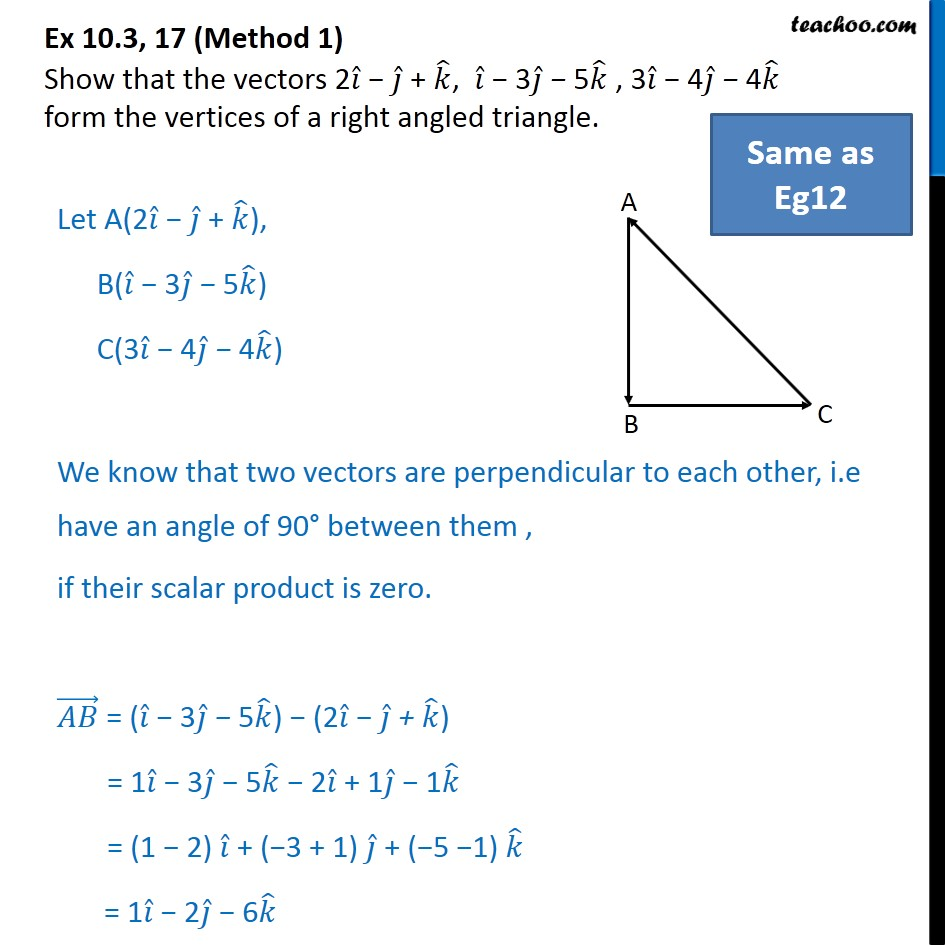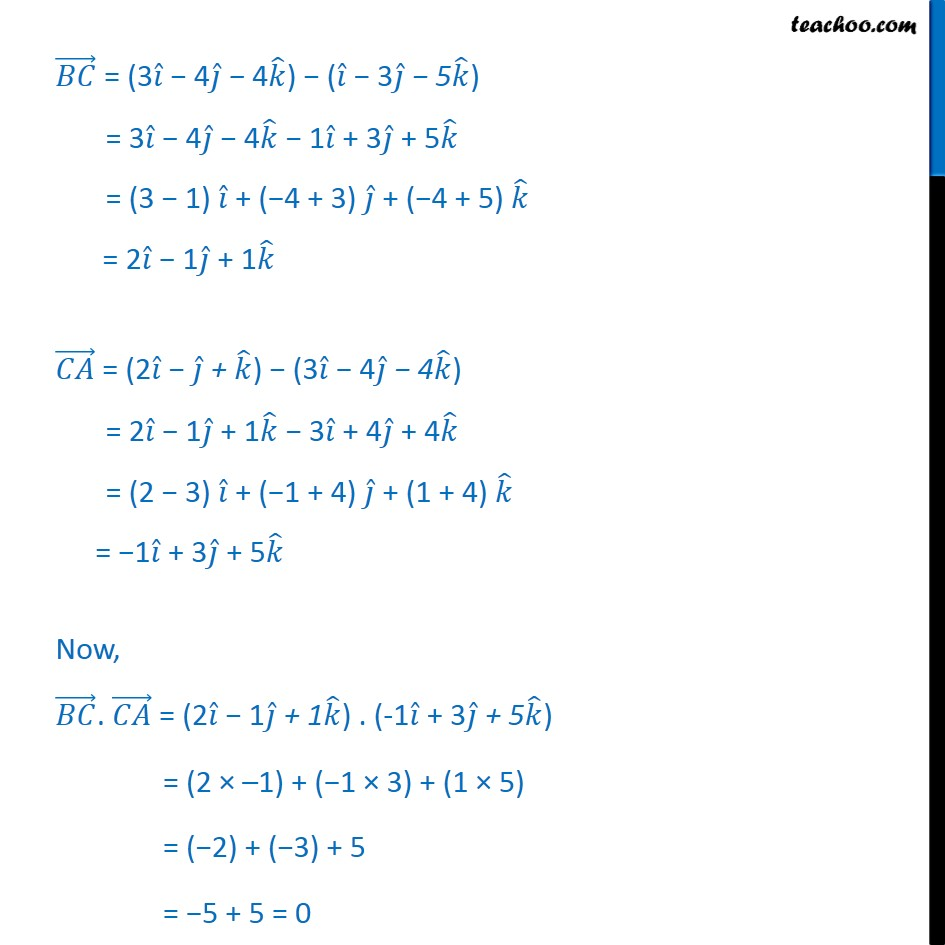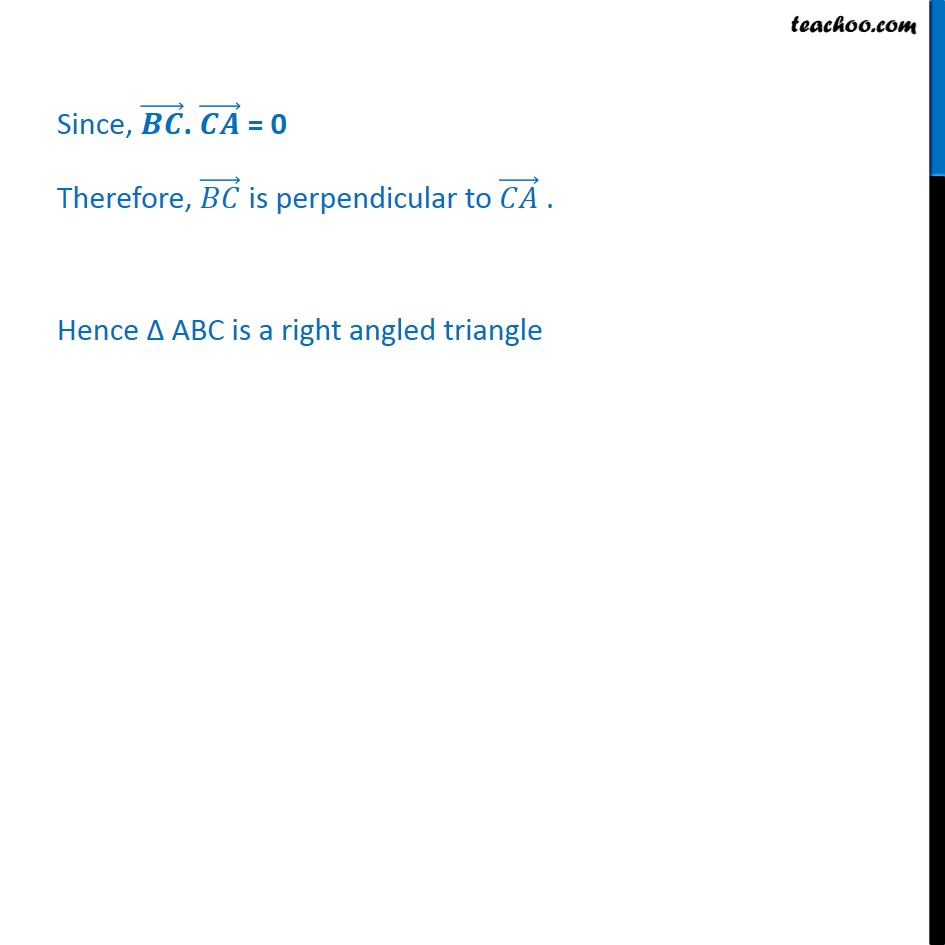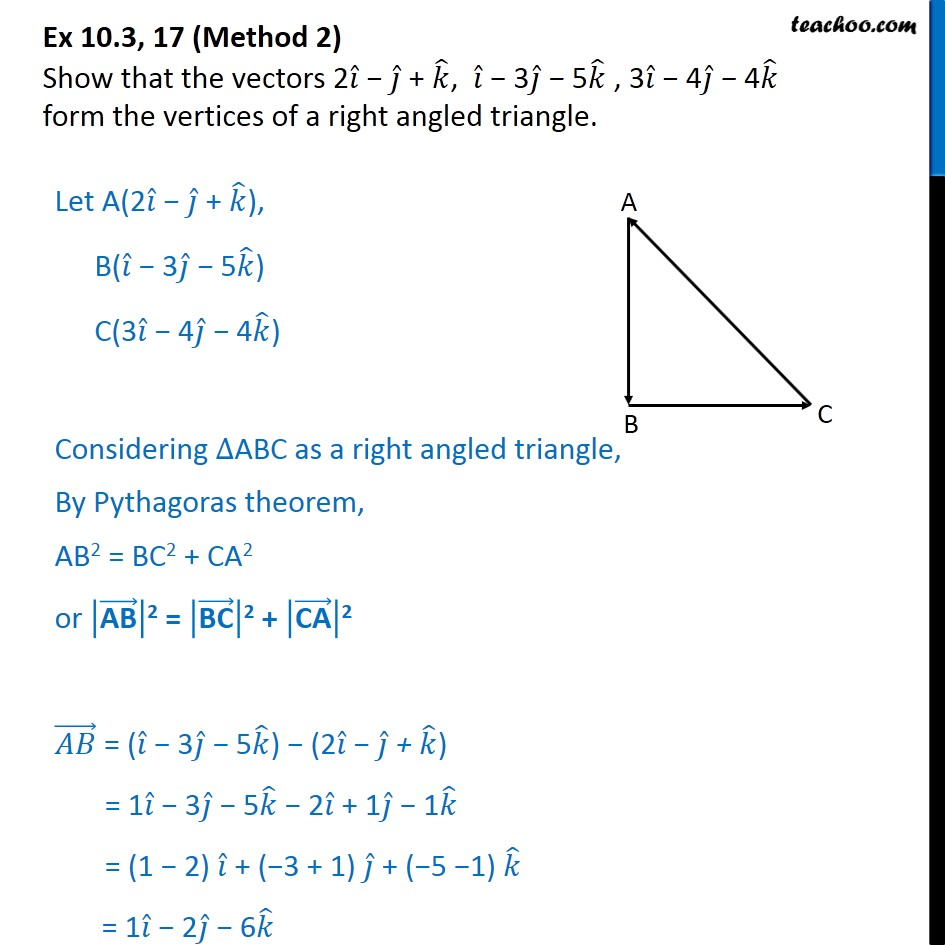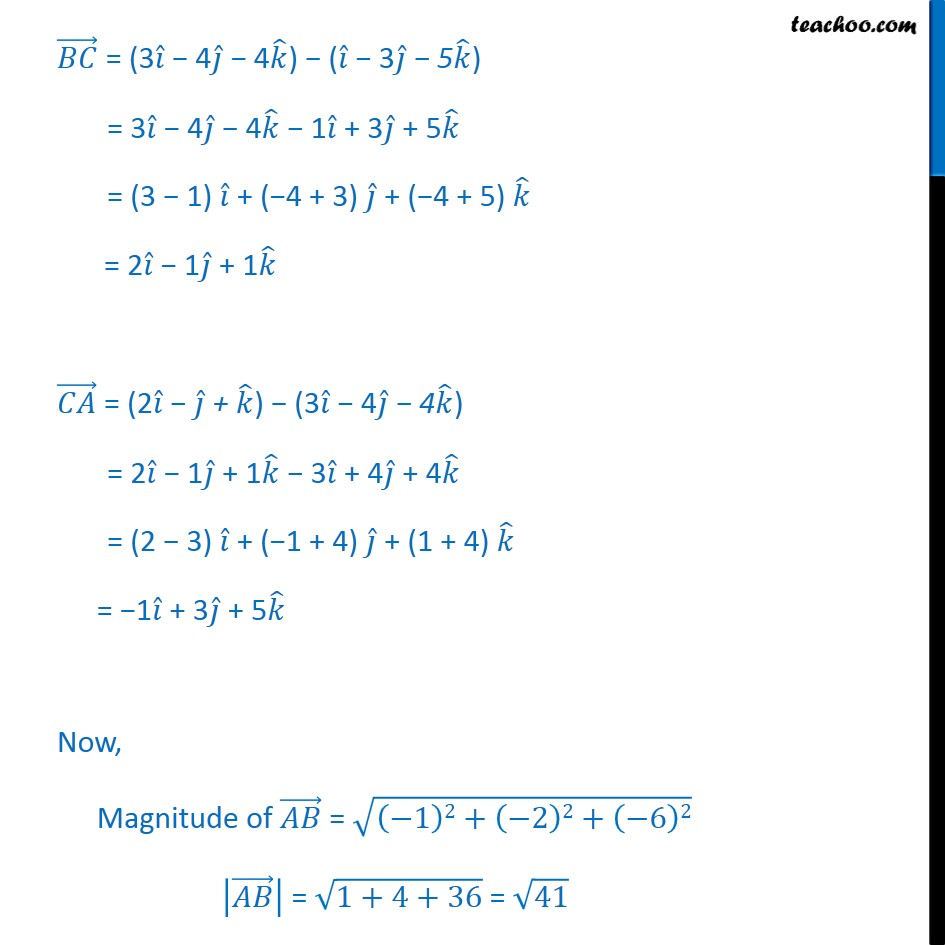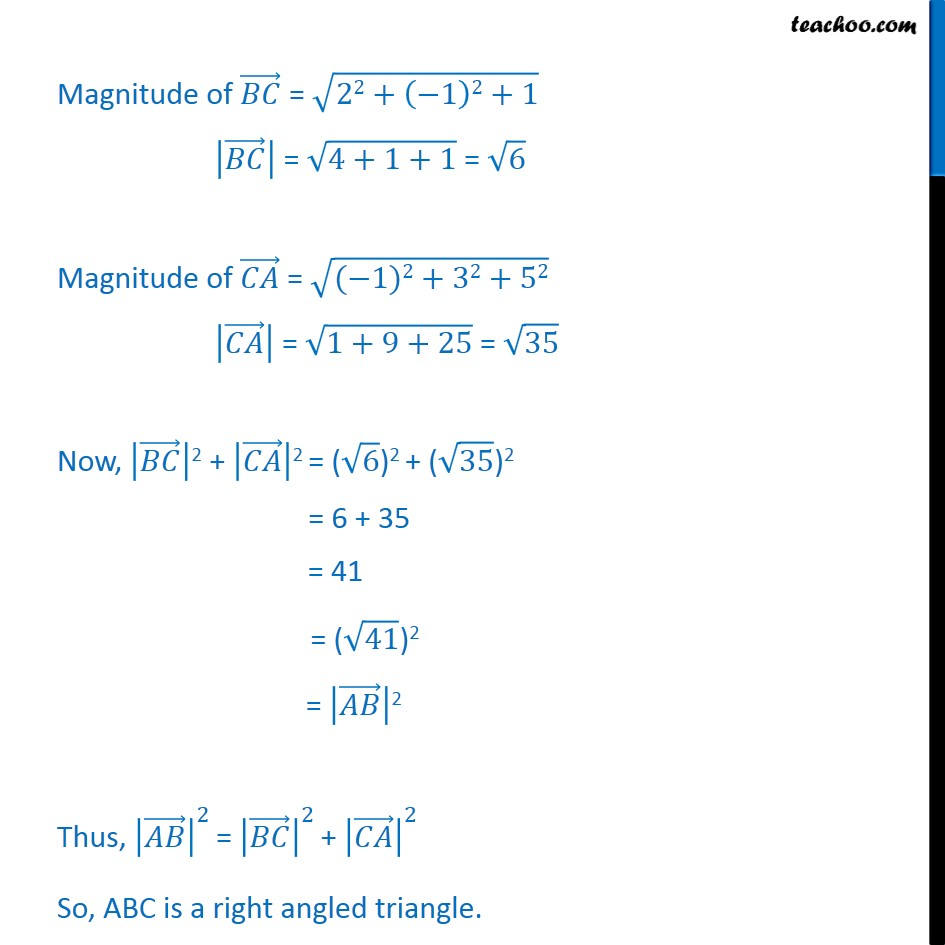1. Chapter 10 Class 12 Vector Algebra
2. Serial order wise
3. Ex 10.3

Transcript

Ex 10.3, 17 (Method 1) Show that the vectors 2 + , 3 5 , 3 4 4 form the vertices of a right angled triangle. Let A(2 + ), B( 3 5 ) C(3 4 4 ) We know that two vectors are perpendicular to each other, i.e have an angle of 90 between them , if their scalar product is zero. = ( 3 5 ) (2 + ) = 1 3 5 2 + 1 1 = (1 2) + ( 3 + 1) + ( 5 1) = 1 2 6 = (3 4 4 ) ( 3 5 ) = 3 4 4 1 + 3 + 5 = (3 1) + ( 4 + 3) + ( 4 + 5) = 2 1 + 1 = (2 + ) (3 4 4 ) = 2 1 + 1 3 + 4 + 4 = (2 3) + ( 1 + 4) + (1 + 4) = 1 + 3 + 5 Now, . = (2 1 + 1 ) . (-1 + 3 + 5 ) = (2 1) + ( 1 3) + (1 5) = ( 2) + ( 3) + 5 = 5 + 5 = 0 Since, . = 0 Therefore, is perpendicular to . Hence ABC is a right angled triangle Ex 10.3, 17 (Method 2) Show that the vectors 2 + , 3 5 , 3 4 4 form the vertices of a right angled triangle. Let A(2 + ), B( 3 5 ) C(3 4 4 ) Considering ABC as a right angled triangle, By Pythagoras theorem, AB2 = BC2 + CA2 or AB 2 = BC 2 + CA 2 = ( 3 5 ) (2 + ) = 1 3 5 2 + 1 1 = (1 2) + ( 3 + 1) + ( 5 1) = 1 2 6 = (3 4 4 ) ( 3 5 ) = 3 4 4 1 + 3 + 5 = (3 1) + ( 4 + 3) + ( 4 + 5) = 2 1 + 1 = (2 + ) (3 4 4 ) = 2 1 + 1 3 + 4 + 4 = (2 3) + ( 1 + 4) + (1 + 4) = 1 + 3 + 5 Now, Magnitude of = 1 2+ 2 2+ 6 2 = 1+4+36 = 41 Magnitude of = 22+ 1 2+1 = 4+1+1 = 6 Magnitude of = ( 1)2+32+52 = 1+9+25 = 35 Now, 2 + 2 = ( 6 )2 + ( 35 )2 = 6 + 35 = 41 = ( 41 )2 = 2 Thus, 2 = 2 + 2 So, ABC is a right angled triangle.

Ex 10.3